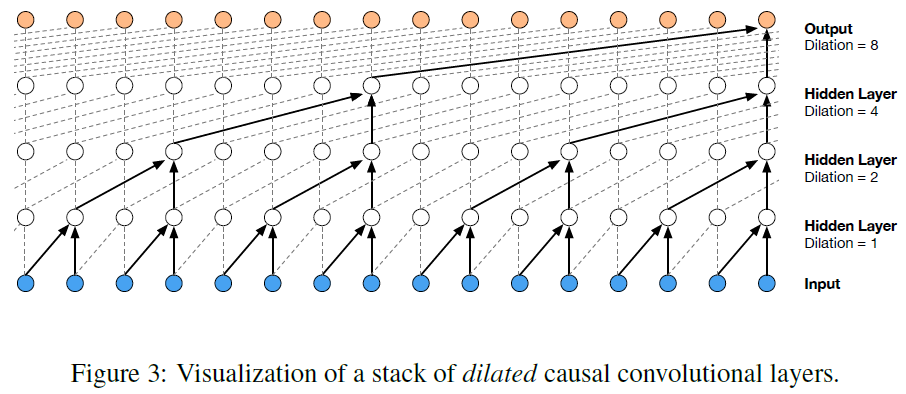# Chapter 15 Processing Sequences Using RNNs and CNNs

Chapter 15  Processing Sequences Using RNNs and CNNs

Hands-On Machine Learning with Scikit-Learn, Keras & Tensorflow 2nd Edition by A. Geron

RNNs are not the only types of neural networks capable of handling sequential data:

for small sequences, a regular dense network can do the trick:

and for very long sequences, such as audio samples or text,

convolutional neural networks can actually work quite well too.

We will discuss both of these possibilities, and we will finish this chapter by implementing a WaveNet: this is a CNN architecture capable of handling sequences of tens of thousands of time steps.

In Chapter 16, we will continue to explore RNNs and see how to use for natural language processing, along with more recent architectures based on attention mechanisms.

Let's get started.

RNNを使った自然言語処理は、次の章で説明する。しかも、attention mechanismをベースにして！

dense networkでも短文なら扱えるし、非常に長い文章や音声データならCNNでも高い性能を発揮するし、最後に実装する音声変換モデルWaveNetもCNNである。

Recurrent Neurons and Layers

Mamory Cells

Input and Output Sequences

Training RNNs

Forcaasting a Time Series

Implementing a simple RNN

Trend and Seasonality

Deep RNNs

Handling Long Sequences

Tackling the Short-Term Memory Problem

LSTM cells

Peephole connections

GRU cells

Using 1D convolutional layers to process sequences

In Chapter 14, we saw that a 2D convolutional layer works by sliding several fairly small kernels (or filters) across an image, producing multiple 2D feature maps (one per kernal).

Similarly, a 1D convolutional layer slides several kernels across a sequence, producing a 1D feature map per kernel.

Each kernel will learn to detect a single very short sequential pattern (no longer than the kernal size).

If you use 10 kernels, then the layer's output will be composed of 10 1-dimensional sequences (all of the same length), or equivalently you can view this output as a single 10-dimensional sequence.

This means that you can build a neural network composed of a mix of recurrent layers and 1D convolutional layers (or even 1D pooling layers).

If you use a 1D convolutional layer with a stride of 1 and "same" padding, then the output sequence will have the same length as the input sequence.

But if you use "valid" padding or a stride greater than 1, then the output sequence will be shorter than the input sequence, so make sure you adjust the targets accordingly.

For example, the following model is the same as earlier, except it starts with a 1D convolutional layer that downsamples the input sequence by a factor of 2, using a stride of 2.

The kernal size is larger than the stride, so all inputs will be used to compute the layer's output, and therefore the model can learn to preserve the useful information, dropping only the unimportant details.

By shortning the sequences, the convolutional layer may help the GRU layers detect longer patterns.

Note that we must also crop off the first three time steps in the targets (since the kernel's size is 4, the first output of the convolutional layer will be based on the input time steps 0 to 3), and downsample the targets by a factor of 2:

WaveNet

In a 2016 paper, Aaron van den Oord and other Deep-Mind researchers introduced an architecture called WaveNet.

They stacked 1D convolutional layers, doubling the dilation rate (how spread apart each neuron's inputs are) at each layer:

the first convolutional layer gets a glimpse of just two time steps at a time, while the next one sees four time steps (its receptive field is four time steps long), the next one sees eight time steps, and so on.This way, the lower layers learn short-term patterns, while the higher layers learn long-term patterns.

Thanks to the doubling dilation rate, the network can process extremely large sequence very efficiently.

In the WaveNet paper, the authors actually stacked convolutional layers with dilation rates of 1, 2, 4, 8, ..., 256, 512, then they stacked another group of 10 identical layers (also with dilation rates 1, 2, 4, 8, ..., 256, 512), then again another identical group of 10 layers.

They justified this architecture by pointing out that a single stack of 10 convolution layers with these dilation rates will act like a super-efficient convolutional layer with a kernel of size 1,024 (ezcept way faster, more powerful, and using significantly fewer parameters), which is why they stacked 3 such blocks.

They also left-padded the input sequences with a number of zeros equal to the dilation rate before every layer, to preserve the same sequence length throughout the network.

Here is how to impliment a simplified WaveNet to tackle the same sequence as earlier:

model = keras.models.Sequential( )

for rate in (1, 2, 4, 8) * 2:

activation="relu", dilation_rate=rate))

history = model.fit(X_train, Y_train, epochs=20,

validation_data=(X_valid, Y_valid))

This Sequential model starts with an explicit input layer (this is simpler than trying to set input_shape only on the first layer), then continues with a 1D convolutional layer using "causal" padding:

this ensures that the convolutional layer does not peek into the future when making predictions (it is equivalent to padding the inputs with the right amount of zeros on the left and using "valid" padding).

We then add similar pairs of layers using growing dilation rates: 1, 2, 4, 8, and again 1, 2, 4, 8.

Finally, we add the output layer: a convolutional layers with 10 filters of size 1 and without any activation function.

Thanks to the padding layers, every convolutional layer outputs a sequence of the same length as the input sequences, so the targets we use during training can be the full sequences:

no need to crop them or downsample them.

WaveNetが気になったので、最後の2節を先に覗いてみたが、15章全体が、同じデータベースを用いることによって、各モデルの性能を比較してきているので、最初から読まないとだめだとわかった。（5月25日）